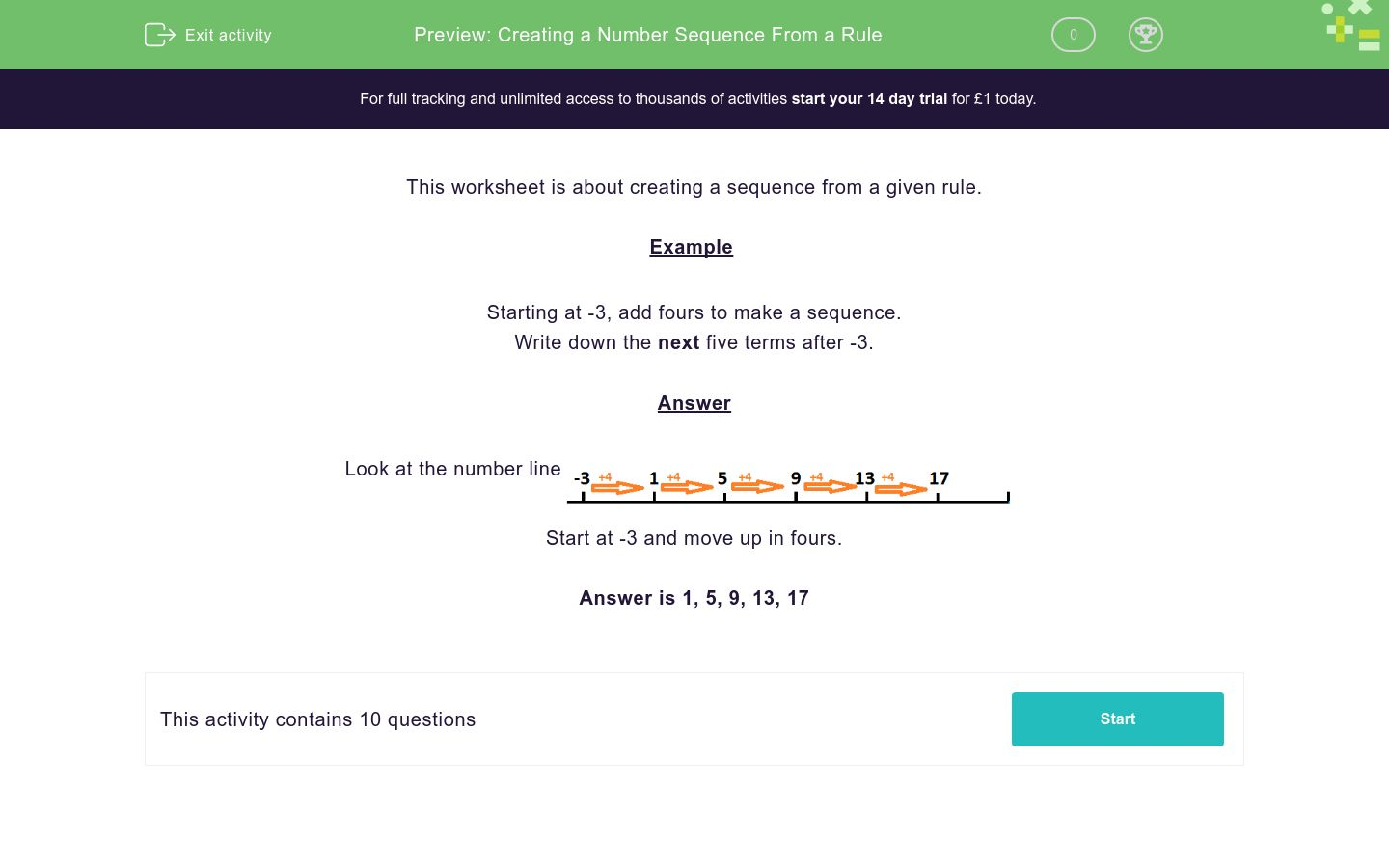# Creating a Number Sequence From a Rule

In this worksheet, students are given a rule from which they create a number sequence. The sequence sometimes include negative numbers.Key stage:  KS 2

Curriculum topic:   Number: Number and Place Value

Curriculum subtopic:   Interpret Negative Numbers

Difficulty level:### QUESTION 1 of 10

This worksheet is about creating a sequence from a given rule.

Example

Starting at -3, add fours to make a sequence.

Write down the next five terms after -3.

Look at the number lineStart at -3 and move up in fours.

Answer is 1, 5, 9, 13, 17

Starting at 23, add fives to make a sequence.

Write down the next five terms after 23.

Starting at 18, add fives to make a sequence.

Write down the next five terms after 18.

Starting at 18, add fours to make a sequence.

Write down the next five terms after 18.

Starting at 15, add sixes to make a sequence.

Write down the next five terms after 15.

Starting at 23, add sevens to make a sequence.

Write down the next five terms after 23.

Starting at -3, add fives to make a sequence.

Write down the next five terms after -3.

Starting at 86, add fives to make a sequence.

Write down the next five terms after 86.

Starting at 29, add eights to make a sequence.

Write down the next five terms after 29.

Starting at 8, add nines to make a sequence.

Write down the next five terms after 8.

Starting at -10, add fives to make a sequence.

Write down the next five terms after -10.

• Question 1

Starting at 23, add fives to make a sequence.

Write down the next five terms after 23.

28
33
38
43
48
EDDIE SAYS
23 + 5 = 28 28 + 5 = 33 33 + 5 = 38 38 + 5 = 43 43 + 5 = 48
• Question 2

Starting at 18, add fives to make a sequence.

Write down the next five terms after 18.

23
28
33
38
43
EDDIE SAYS
18 + 5 = 23 23 + 5 = 28 28 + 5 = 33 33 + 5 = 38 38 + 5 = 43
• Question 3

Starting at 18, add fours to make a sequence.

Write down the next five terms after 18.

22
26
30
34
38
EDDIE SAYS
18 + 4 = 22 22 + 4 = 26 26 + 4 = 30 30 + 4 = 34 34 + 4 = 38
• Question 4

Starting at 15, add sixes to make a sequence.

Write down the next five terms after 15.

21
27
33
39
45
EDDIE SAYS
15 + 6 = 21 21 + 6 = 27 27 + 6 = 33 33 + 6 = 39 39 + 6 = 45
• Question 5

Starting at 23, add sevens to make a sequence.

Write down the next five terms after 23.

30
37
44
51
58
EDDIE SAYS
23 + 7 = 30 30 + 7 = 37 37 + 7 = 44 44 + 7 = 51 51 + 7 = 58
• Question 6

Starting at -3, add fives to make a sequence.

Write down the next five terms after -3.

2
7
12
17
22
EDDIE SAYS
-3 + 5 = 2 2 + 5 = 7 7 + 5 = 12 12 + 5 = 17 17 + 5 = 22
• Question 7

Starting at 86, add fives to make a sequence.

Write down the next five terms after 86.

91
96
101
106
111
EDDIE SAYS
86 + 5 = 91 91 + 5 = 96 96 + 5 = 101 101 + 6 = 106 106 + 5 = 111
• Question 8

Starting at 29, add eights to make a sequence.

Write down the next five terms after 29.

37
45
53
61
69
EDDIE SAYS
29 + 8 = 37 37 + 8 = 45 45 + 8 = 53 53 + 8 = 61 61 + 8 = 69
• Question 9

Starting at 8, add nines to make a sequence.

Write down the next five terms after 8.

17
26
35
44
53
EDDIE SAYS
8 + 9 = 17 17 + 9 = 26 26 + 9 = 35 35 + 9 = 44 44 + 9 = 53
• Question 10

Starting at -10, add fives to make a sequence.

Write down the next five terms after -10.

-5
0
5
10
15
EDDIE SAYS
-10 + 5 = -5 -5 + 5 = 0 0 + 5 = 5 5 + 5 = 10 10 + 5 = 15
---- OR ----

Sign up for a £1 trial so you can track and measure your child's progress on this activity.

### What is EdPlace?

We're your National Curriculum aligned online education content provider helping each child succeed in English, maths and science from year 1 to GCSE. With an EdPlace account you’ll be able to track and measure progress, helping each child achieve their best. We build confidence and attainment by personalising each child’s learning at a level that suits them.

Get started